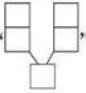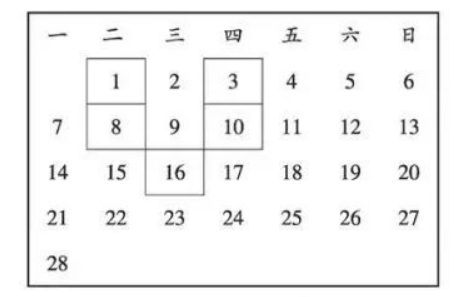(1)在图中用优美的$\mathrm{U}$ 形框框住五个数, 其中最小的数为 1 , 则 $\mathrm{U}$ 形框中的五个数

(2)在图中将 $\mathrm{U}$ 形框上下左右移动, 框住日历表中的 5 个数字, 设最小的数字为 $x$, 用代数 式表示 $U$ 形框框住的五个数字之和为
(3) 在图中移动 $U$ 形框的位置, 框住的五个数字之和可以为 63 吗? 若能, 求出这五个数字 中最小的数; 若不能, 请说明理由.(2) $5 x+33$
(3)设 $\mathrm{U}$ 形框框住的五个数字之和为 63 , 则 $5 x+33=63$, 解得 $x=6$, 由图看出 6 在第 7 列, 所 以框住的五个数字之和不可以为 63 .